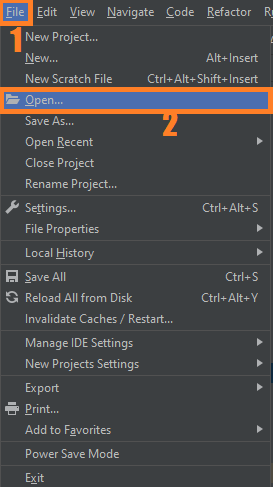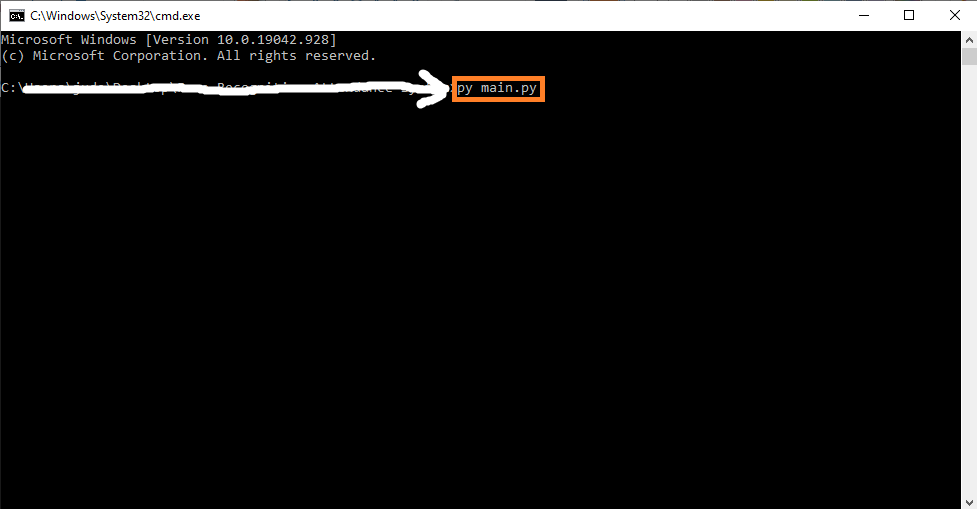# Document Scanner OpenCV Python With Source Code

## Document Scanner OpenCV Python With Source Code

The Document Scanner OpenCV Python was developed using Python OpenCV, The scanner takes a poorly scanned image, finds the corners of the document, applies the perspective transformation to get a top-down view of the document, sharpens the image, and applies an adaptive color threshold to clean up the image.

## What is OpenCV?

OpenCV is short for Open Source Computer Vision. Intuitively by the name, it is an open-source Computer Vision and Machine Learning library. This library is capable of processing real-time image and video while also boasting analytical capabilities. It supports the Deep Learning frameworks.

By the way if you are new to python programming and you don’t know what would be the the Python IDE to use, I have here a list of Best Python IDE for Windows, Linux, Mac OS that will suit for you. I also have here How to Download and Install Latest Version of Python on Windows.

To start executing Document Scanner OpenCV Python With Source Code, make sure that you have installed Python 3.9 and PyCharm in your computer.

## Document Scanner OpenCV Python With Source Code : Steps on how to run the project

Time needed: 5 minutes

These are the steps on how to run Document Scanner OpenCV Python With Source Code

First, download the given source code below and unzip the source code.• Step 2: Import the project to your PyCharm IDE.• Step 3: Run the project.

last, run the project with the command “py main.py”## Installed Libraries

```import numpy as np
import cv2
import imutils```

## Complete Source Code

```import numpy as np
import cv2
import imutils

args_image ="docu.jpg"

image=cv2.resize(image,(500,500))
orig = image.copy()
cv2.imshow("Original Image", image)
cv2.waitKey(0)
cv2.destroyAllWindows()

grayImage = cv2.cvtColor(image, cv2.COLOR_BGR2GRAY)
grayImageBlur = cv2.blur(grayImage,(2,2))
edgedImage = cv2.Canny(grayImageBlur, 100, 300, 3)

cv2.imshow("gray", grayImage)
cv2.waitKey(0)
cv2.destroyAllWindows()
cv2.imshow("grayBlur", grayImageBlur)
cv2.waitKey(0)
cv2.destroyAllWindows()
cv2.imshow("Edge Detected Image", edgedImage)
cv2.waitKey(0)
cv2.destroyAllWindows()

allContours = cv2.findContours(edgedImage.copy(), cv2.RETR_LIST, cv2.CHAIN_APPROX_SIMPLE)
allContours = imutils.grab_contours(allContours)
allContours = sorted(allContours, key=cv2.contourArea, reverse=True)[:1]

perimeter = cv2.arcLength(allContours, True)
ROIdimensions = cv2.approxPolyDP(allContours, 0.02*perimeter, True)
cv2.drawContours(image, [ROIdimensions], -1, (0,255,0), 2)
cv2.imshow("Contour Outline", image)
cv2.waitKey(0)
cv2.destroyAllWindows()
ROIdimensions = ROIdimensions.reshape(4,2)
rect = np.zeros((4,2), dtype="float32")
s = np.sum(ROIdimensions, axis=1)
rect = ROIdimensions[np.argmin(s)]
rect = ROIdimensions[np.argmax(s)]
diff = np.diff(ROIdimensions, axis=1)
rect = ROIdimensions[np.argmin(diff)]
rect = ROIdimensions[np.argmax(diff)]

(tl, tr, br, bl) = rect

widthA = np.sqrt((tl -tr)**2 + (tl - tr)**2 )
widthB = np.sqrt((bl - br)**2 + (bl - br)**2 )
maxWidth = max(int(widthA), int(widthB))

heightA = np.sqrt((tl - bl)**2 + (tl - bl)**2 )
heightB = np.sqrt((tr - br)**2 + (tr - br)**2 )
maxHeight = max(int(heightA), int(heightB))
dst = np.array([
[0,0],
[maxWidth-1, 0],
[maxWidth-1, maxHeight-1],
[0, maxHeight-1]], dtype="float32")

transformMatrix = cv2.getPerspectiveTransform(rect, dst)
scan = cv2.warpPerspective(orig, transformMatrix, (maxWidth, maxHeight))
cv2.imshow("Scaned",scan)
cv2.waitKey(0)
cv2.destroyAllWindows()
scanGray = cv2.cvtColor(scan, cv2.COLOR_BGR2GRAY)
cv2.imshow("scanGray", scanGray)
cv2.waitKey(0)
cv2.destroyAllWindows()
from skimage.filters import threshold_local
T = threshold_local(scanGray, 9, offset=8, method="gaussian")
scanBW = (scanGray > T).astype("uint8") * 255
cv2.imshow("scanned", scanBW)
cv2.waitKey(0)
cv2.destroyAllWindows()```